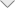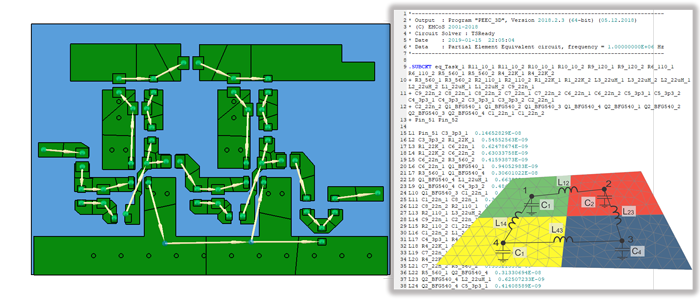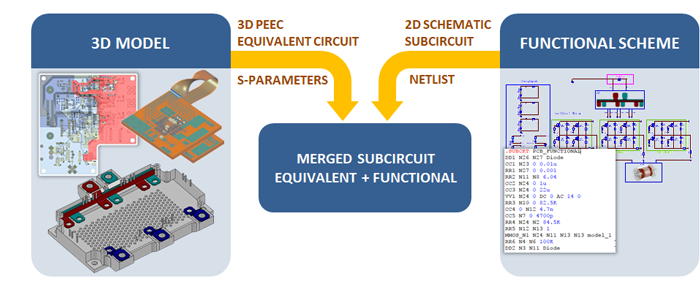### Computer Simulation Software

> > > > 3D PEEC Solver

### 3D PEEC Solver

3D PEEC solver supports calculation of partial inductance (L), resistance (R), capacitance (C) and conductance (G) of 3-dimensional metal-dielectric objects, generation of Partial Element Equivalent Circuit (PEEC) and calculation of corresponding S-parameters matrix.

To calculate mutual and self-inductances and resistances of branches, the 3D MoM-based Low Frequency Magnetic Field solver (LFMF) is used. 3D MoM-based quasi-electrostatic solver (LFEF) is applied to calculate mutual and self-capacitances of nodes.After all RLCG parameters are calculated, PEEC model is represented with lumped elements in SPICE format circuit file.

The PEEC circuit can be combined with functional model and then calculated using MNA. In practice, the functional circuit and PEEC model coupling procedure yields quite a complex circuit  topology. The fast circuit simulation demand was fulfilled by developing a speed-oriented circuit solver, capable of simulating very large mixed networks consisting of millions of elements.﻿